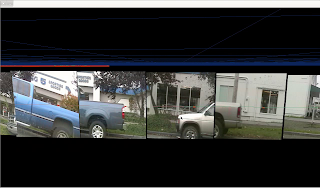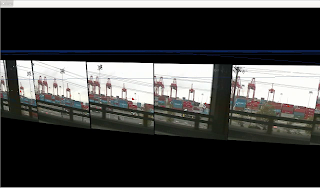## 2013-10-24

### Software Archaeology #1: GPS tagged street video

Around 10 years ago I was working on a number of personal software projects with a mostly common C++ code-base that had a lot of boilerplate OpenGL and vector classes I'd built up from reading the NeHe tutorials.  Some of that work was properly documented and put into source control and madee public, the rest were periodically made into version numbered tarballs.  When I finished or lost interest in developing some graphics technique or physics simulation or anything else I would rename the directory to reflect the new project and start on new functionality: some of old was still useful, some of it had to get ifdeffed out, and some just sat unused.  Some of those were documented but not open-sourced, and a few of those tarballs were archived in my online home directory.  Eventually a lot of the code was superseded by vastly superior open source libraries so it didn't make sense to continue using it, but I would sometimes make backups of the old stuff on DVD and copy them to multiple hard drives as I bought them but with less and less care as time went by.

Fast forward to the present, and reading a section of Planet Google about StreetView, and I started thinking about a particular project where I was driving around Seattle with a DV camera mounted in on the passenger side and a GPS on my roof being logged on a laptop.  I'm pretty sure I was inspired by reading about the Aspen Movie Map from the +Howard Rheingold book Virtual Reality.

Some OpenGL software loaded the images extracted from the video and then displayed them on top of a 3D GPS trajectory.  It worked fine, but I only did it once and took no screenshots or videos and told no more than one or two people about it.  Maybe I thought it was a such a good idea it had to be kept secret until the opportunity to capitalize arose, obviously the opportunity is now long past.  But it it still was fun to have done and having it run again would be cool... but I couldn't find it on any of my still running desktop computers or laptops.  Eventually I found a 250GB Maxtor drive in a shoebox and plugged it in with a usb-to-sata adapter, and there it was: 700 megabytes of video and images all nicely organized along with scripts and source code.  And it compiled: after resolving the SDL dependencies the only thing I had to do was move the ordering  -lGL etc. linker options to be after the listing of object files:    \$(CXX) -o \$(PROGRAM) \$(OBJECTS) \$(LIBS) instead of   \$(CXX) -o \$(PROGRAM)  \$(LIBS) \$(OBJECTS).  And it ran fine with ./gpsimage --gps ../capture_10_22_2004.txt --bmp biglist.txt, and with some minor modification to the keyboard controls and the resolution I was able to take screenshots and a video:Ballard surface streetsBallard surface streetsExiting the tunnel to get on the viaductDriving south on the 99 viaduct looking west

### Implementation

It might be nice to actually check in some of the code to github or something, but for now I'll document the important parts here.

I used dvgrab to extract video from the camera, and converted that to decimated timestamped bmp images.  The text gps log which looks like this:

\$GPGGA,162651.395,4740.2379,N,12222.4207,W,1,06,1.5,15.0,M,-17.3,M,0.0,0000*7E
\$GPGSA,A,3,23,13,16,20,01,25,,,,,,,2.8,1.5,2.4*3A
\$GPGSV,3,1,09,23,81,041,46,13,51,298,48,16,46,083,46,20,42,175,44*7F
\$GPGSV,3,2,09,01,20,100,37,04,19,284,34,27,19,240,40,25,16,061,40*7E
\$GPGSV,3,3,09,24,12,320,30*47
\$GPRMC,162651.395,A,4740.2379,N,12222.4207,W,22.57,179.63,221004,,*2C
\$GPGGA,162652.395,4740.2316,N,12222.4208,W,1,06,1.5,14.4,M,-17.3,M,0.0,0000*7E
\$GPGSA,A,3,23,13,16,20,01,25,,,,,,,2.8,1.5,2.4*3A
\$GPRMC,162652.395,A,4740.2316,N,12222.4208,W,22.64,178.75,221004,,*2F
\$GPGGA,162653.395,4740.2253,N,12222.4208,W,1,06,1.5,13.8,M,-17.3,M,0.0,0000*74
\$GPGSA,A,3,23,13,16,20,01,25,,,,,,,2.8,1.5,2.4*3A
\$GPRMC,162653.395,A,4740.2253,N,12222.4208,W,22.76,178.28,221004,,*25
\$GPGGA,162654.395,4740.2189,N,12222.4208,W,1,06,1.5,13.2,M,-17.3,M,0.0,0000*7D
\$GPGSA,A,3,23,13,16,20,01,25,,,,,,,2.8,1.5,2.4*3A
...

was converted like this:

ifstream parts(fileName.c_str());
if (!parts) {
exit(1);
}

vector3f initialPos;
string lines;
while (getline(parts,lines)) {
//cout << lines << "\n";
vector<string> tokens = tokenize(lines,",");

if ((tokens.size() > 0) && (tokens == "\$GPGGA") && tokens.size() > 9) {

float rawTime = atof(tokens.c_str());

int tsec = (int)rawTime%100;
int tmin = ((int)rawTime/100)%100;
/// convert to local time
int thr = (int)rawTime/10000 -7;
float time =  (float)thr + ((float)tmin+tsec/60.0f)/60.0f;

vector3f pos = vector3f(10000.0f*atof(tokens.c_str())-initialPos,
atof(tokens.c_str())-initialPos,
-10000.0f*atof(tokens.c_str())- initialPos
);

if (initialPos == vector3f()) {
initialPos = pos;
pos = vector3f(0,0,0);
}

pair<float,vector3f> tp(time,pos);
timePos.push_back(tp);

}

}

(tokenize was a function to split up lines of text, I think the standard C++ libraries didn't do that at the time)

The timestamped bmp files look like this in a directory:

vid_2004.10.20_09-24-49.bmp
vid_2004.10.20_09-24-50.bmp
vid_2004.10.20_09-24-51.bmp
vid_2004.10.20_09-24-52.bmp
vid_2004.10.20_09-24-53.bmp
vid_2004.10.20_09-24-54.bmp
...

ifstream bmpList(bmpListFileName.c_str());
if (!bmpList) {
exit(1);
}

while (getline(bmpList,lines)) {

vector<string> tokens = tokenize(lines,".");

if (tokens.size() > 3) {
string messyTime = tokens[tokens.size()-2];
vector<string> items = tokenize(tokenize(messyTime,"-"),"_");

if (items.size() == 4) {
//OUT( items << ":" << items << ":" << items);
float time = atof(items.c_str())
+(atof(items.c_str())
+(atof(items.c_str())/60.0f))/60.0f;

/// arbitrary offset to match gps to images better
time += .012f;
timeImage.push_back(pair<float,string>(time,lines));
} else {
OUT("list time wrongly formatted " << messyTime);
}

} else {
OUT("list items have wrong format" << lines);
}
}

Then brute force O(n^2) the correspondence between image timestamps and gps timestamps:

/// using the times extracted from the bmp file names, find what the closest
/// gps coordinates for those times
for (unsigned i = 0; i < timeImage.size(); i++) {
for (unsigned j = 0; j < timePos.size()-1; j++) {
if ((timePos[j].first <= timeImage[i].first)
&& (timePos[j+1].first > timeImage[i].first)) {
struct tpi newTpi;
newTpi.time = timeImage[i].first;
/// interpolate - is this working?
float factor = (newTpi.time - timePos[j].first)
/ (timePos[j+1].first - timePos[j].first);
//OUT(i << " " <<j << " " <<factor);
newTpi.pos = timePos[j].second
+ (timePos[j+1].second - timePos[j].second) * factor;

createTexture(newTpi.texture, timeImage[i].second);

/// don't interpolate just use the same point
//newTpi.pos = timePos[j].second;

/// attitude
vector3f up = vector3f(0,1.0f,0);
/// this is arbitrary based on the fact the video was shot at a right angle to
/// the direction of travel
vector3f right = (timePos[j+1].second - timePos[j].second);
right = right/right.Length();

// make all axes orthogonal
vector3f out = Cross(up,right);
up = Cross(right,out);

// normalize
out   = out/out.Length();
up    = up/up.Length();
newTpi.attitude.Set(right,up,out);

/// scale
if (i >0) {
newTpi.scale = (newTpi.pos - tpiList[i-1].pos).Length()/2.0f;
} else {
newTpi.scale = 5.0f;
}

tpiList.push_back(newTpi);
}
}
}

And then draw it later:

void gps::draw()
{
/// the gps signal
glPushAll();
glColor3f(0.67398f,.459f, 0.459f);
glBegin(GL_LINE_STRIP);
for (unsigned i = 0; i <timePos.size(); i++) {
/// subtract first position to make path always start from origin
glVertex3fv((timePos[i].second).vertex);
}
glEnd();
glColor3f(0.67398f,.159f, 0.059f);
glPointSize(9.0f);
glBegin(GL_POINTS);
for (unsigned i = 0; i <timePos.size(); i++) {
/// subtract first position to make path always start from origin
glVertex3fv((timePos[i].second).vertex);
}
glEnd();

/// interpolated image position
glColor3f(0.37398f,.659f, 0.459f);
glBegin(GL_LINE_STRIP);
for (unsigned i = 0; i <tpiList.size(); i++) {
glVertex3fv((tpiList[i].pos).vertex);
}
glEnd();
/*  glColor3f(0.17398f,0.559f, 0.859f);
glPointSize(10.0f);
glBegin(GL_POINTS);
for (unsigned i = 0; i <tpiList.size(); i++) {
glVertex3fv((tpiList[i].pos).vertex);
}
glEnd();
*/
glPopAll();

glPushAll();

glEnable(GL_TEXTURE_2D);
glColor3f(1.0f,1.0f,1.0f);

/// always pointed at camera
//matrix16f temp = Registry::instance()->theCamera->location;
//temp.SetTranslation(vector3f(0.0f,0.0f,0.0f));

vector3f loc = Registry::instance()->theCamera->location.GetTranslation();

int oldI = 0;
for (unsigned i = 0; i <tpiList.size(); i++) {
float scale = tpiList[i].scale;

/// simple distance culling
float dist = (loc - tpiList[i].pos).Length();
/*if ((dist >= 5000)) {
/// make far away textures bigger, and show less of them
float f= dist/5000;
f =f*f;
i += (int)f+1;
scale*= f;
}*/
if ((dist > 3000) && (dist <= 8000)) {
if (i%5==0) {
//i+=10;
scale *=5;
} else {
dist = 20000;
}
}
if (dist > 8000) {
if (i%10==0) {
//i+=10;
scale *=10;
} else {
dist = 20000;
}
}
if (dist < 16000) {
glBindTexture(GL_TEXTURE_2D, tpiList[i].texture);

matrix16f temp = tpiList[i].attitude;
glTexCoord2f(0.0f, 0.0f);
glVertex3fv((tpiList[i].pos+temp.Transform(scale*vector3f(1.0,1.0,0.0))).vertex);
glTexCoord2f(1.0f, 0.0f);
glVertex3fv((tpiList[i].pos+temp.Transform(scale*vector3f(-1.0,1.0,0.0))).vertex);
glTexCoord2f(1.0f, 1.0f);
glVertex3fv((tpiList[i].pos+temp.Transform(scale*vector3f(-1.0,-1.0,0.0))).vertex);
glTexCoord2f(0.0f, 1.0f);
glVertex3fv((tpiList[i].pos+temp.Transform(scale*vector3f(1.0,-1.0,0.0))).vertex);

glEnd();
}
oldI = i;
}

glPopAll();

}

### Future

A few other old projects could be revived, though some have more obscure dependencies (paragui and maybe another opengl gui).  It's not a high priority but it would be nice to create better records now than wait even longer for more bitrot to set in, and I have a restored interest in low-ish level OpenGL so it would be nice to get refreshed on the stuff I've already done.